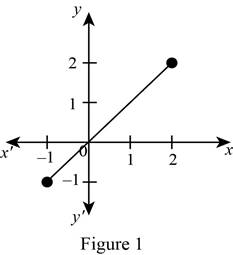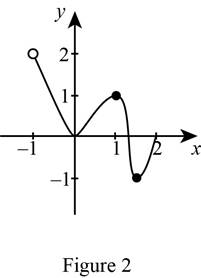# The graph of a function on [ − 1 , 2 ] that has an absolute maximum but no local maximum.### Single Variable Calculus: Concepts...

4th Edition
James Stewart
Publisher: Cengage Learning
ISBN: 9781337687805### Single Variable Calculus: Concepts...

4th Edition
James Stewart
Publisher: Cengage Learning
ISBN: 9781337687805

#### Solutions

Chapter 4.2, Problem 12E

(a)

To determine

## To sketch: The graph of a function on [−1,2] that has an absolute maximum but no local maximum.

Expert Solution

### Explanation of Solution

Let x be represented in the x-axis and the value of the function f(x) be represented in the y-axis.

Absolute maximum is any point of the domain at which the function attains its maximum value. Since the given interval is [1,2] , choose the maximum point at x = 2.

Local maximum is the point in the considered interval of the domain at which the function attains its maximum value.

Draw the graph of the function f in such a way that it satisfies the given conditions as shown below in Figure 1.From Figure 1, observe that the absolute maximum occurs at x=2 . Since local extrema cannot occur at end points, the graph has no local maximum.

(b)

To determine

Expert Solution

### Explanation of Solution

Let x be represented in the x-axis and the value of the function f(x) be represented in the y-axis.

Local maximum is the point in the considered interval of the domain at which the function attains its maximum value.

Consider any greatest point on the interval [1,2] but should not consider the end points as the local maximum does not at end points. Hence, consider the point (1, 1).

Absolute maximum is any point of the domain at which the function attains its maximum value.

Draw the graph of the function f in such a way that it satisfies the given conditions as shown below in Figure 2.From Figure 2, it is observed that the graph has local maximum at x=1 . Since the curve has hole at x=1 , the point is not included in the domain. Therefore, it has no absolute maximum at x=1 .

Note: If it has no hole at x=1 then absolute maximum occurs at x=1 .

### Have a homework question?

Subscribe to bartleby learn! Ask subject matter experts 30 homework questions each month. Plus, you’ll have access to millions of step-by-step textbook answers!